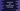# JavaScript program to get unique values in an arrayIn this JavaScript tutorial, we will learn how to get unique values from an array of different elements. The input of the program is an array and the output of the program is also an array containing only the unique elements of that array.

## Using Set :

A set can hold only unique values. If we pass an array to the set constructor, it transforms that array to a set removing all duplicate values. Again, by using a spread operator, we can convert that set to an array.

``````let arr = [1,2,3,'a','b','c','a',2,3]

let arrSet = new Set(arr)

let newArr = [...arrSet]

console.log(newArr)``````

It will print :

``[ 1, 2, 3, 'a', 'b', 'c' ]``

We can also write the above program in short :

``````let arr = [1,2,3,'a','b','c','a',2,3]

let newArr = [...new Set(arr)]

console.log(newArr)``````

## Using filter :

Another way to remove the unique values from an array is by using filter. This method takes one callback function and filter out elements based on the logic we write in this function.

If I write the above program using filter, it looks as like below :

``````let givenArr = [1,2,3,'a','b','c','a',2,3]

let newArr = givenArr.filter((val,i,arr)=> arr.indexOf(val) === i)

console.log(newArr)``````

The callback function takes three params :

• val is the current value
• i is the current item index
• arr is the given array

This callback function is called on each element of the array. We are checking if the index of the item is equal to the current index or not, i.e. if we have two similar elements, it will fail for one element. indexOf returns the first index of an element in an array.

This program will print similar output as the above program.